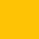TradingView4178 views
Introducing the Delta-RSI Oscillator.

This oscillator is a time derivative of the RSI , plotted as a histogram and serving as a momentum indicator . The derivative is calculated explicitly by means of local polynomial regression. It is designed to provide minimum false and premature buy/sell​ signals compared to many traditional momentum indicators such as Momentum, RSI , Rate of Change .

Application:
Potential trading signals provided by the Delta-RSI Oscillator include:
- zero crossing (negative-to-positive as a bullish sign and positive-to-negative sign as a bearish signal),
- change of direction (consider going long if the oscillator starts to advance, and short otherwise).
In addition, the strength of a particular trend can be estimated by looking at the Delta-RSI value (positive D-RSI in case of the uptrend, and negative in case of the downtrend).

Choosing the model Parameters:
-RSI Length: The timeframe of the RSI that is being differentiated.
- Frame Length: The length of the lookback frame used for local regression.
- Polynomial Order: The order of the local polynomial function.
Longer frames and lower order of polynomials will result in a "smoother" D-RSI, but at the expense of greater lag. Increasing the polynomial order while maintaining the frame length will reduce lag while producing more variance. The values set as default (Length=18, Order=2) were found to provide optimum the variance/lag tradeoff. However, other options (e.g., Length=35, Order=3) can also work well.

Relationship with other methods:
When developing this indicator, I was inspired by​ Connie Brown’s Derivative Oscillator. The latter pursues the same goal but evaluates the RSI derivative by means of triple smoothing. This paves the way for more clear interpretation and easier tuning of model parameters.

リリースノート: Added the Signal Line to serve as ​a possible confirmation of the D-RSI direction change (analogous to the MACD signal line)
リリースノート: .
オープンソーススクリプト

TradingViewの精神に則り、このスクリプトの作者は、トレーダーが理解し検証できるようにオープンソースで公開しています。作者に敬意を表します！無料で使用することができますが、このコードの公開物での再利用には、ハウスルールに準拠する必要があります。お気に入りに登録してチャート上でご利用頂けます。

チャートでこのスクリプトを利用したいですか？

## コメントThis is awesome! Thanks!
Can I use part of your code on my own indicator?This is definitly one of the most unique buts of pine i have come across. Lots to learn from here. Thanks for sharing publicly!